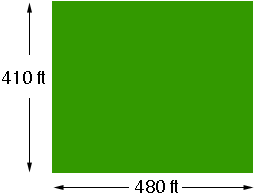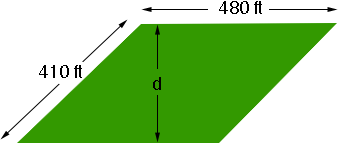SEARCH HOMEMath Central Quandaries & QueriesQuestion from Phyllis: Could you please figure out the square footage and acreage of my lot? One side is 480ft One side is 410ft One side is 480ft The last side is 410ft Thank you.Hi Phyllis,

If the corners are right angles then the area is 480410 = 196800 square feet.If the corners are not square then you need to find the distance d in feet between a pair of sides as in the diagram below.In this diagram, since d is the distance between the sides of length 480 ft the area is 480d square feet.

One acres is 43560 square feet so once you have the area in square feet divide by 43560 to find the area in acres.

PennyMath Central is supported by the University of Regina and The Pacific Institute for the Mathematical Sciences.